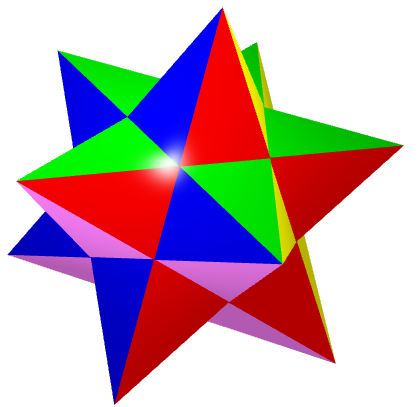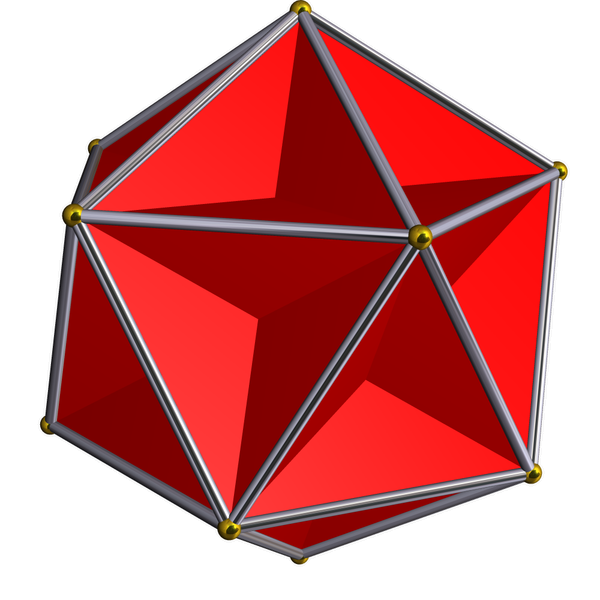# 1.- Introduction

In three dimensions, stellation means constructing a new polyhedron from an existing one by a process of extending elements such as edges or faces, usually in a symmetrical way, until they meet to form a new polygon or polyhedron. The new figure is a stellation of the original. This definition was given by Kepler back in 1619. He stellated the dodecahedron to obtain two of the regular star polyhedra (or Kepler-Poinsot polyhedra). He also stellated the octahedron obtaining the stella octangula or stellated octahedron.

# 2.- Stellated octahedron

The stellated octahedron can be seen either as a polyhedron compound or as a stellation. As a compound, it is constructed as the union of two tetrahedra (a tetrahedron and its dual tetrahedron). The vertices of the resulting polyhedron coincide with the vertexs of a cube (Figure 1a). The intersection of the two tetrahedra form an inner octahedron (Figure 1b) which share the same face-planes with the compound. As a stellation, it is constructed starting from an octahedron by adding small tetrahedra on each face.(a) (b) Figure 1: Stellated octahedron and its inner grid (click on the left image to get a 3D view that you can zoom and rotate).

The stellated octahedron is at the core of the inner grid of Metatron's Cube, which can be constructed by making a square of four such polyhedra, and another square on top of it. See our article on Metatron's Cube for a detailed description.

# 3.- The Kepler-Poinsot polyhedra

These polyhedra can be obtained by stellating the dodecahedron and the isosahedron. They have pentagrams as faces or vertex figures. The great dodecahedron and great icosahedron have convex polygonal faces, but pentagrammic vertex figures. The small and great stellated dodecahedron have nonconvex regular pentagram faces. The Kepler-Poinsot polyhedra exist in dual pairs: the small stellated dodecahedron is the dual of the great dodecahedron, and the great stellated dodecahedron is the dual of the great icosahedron (Figure 2).

SOLID DUAL SOLID(a) Great Dodecahedron (b) Small Stellated Dodecahedron(c) Great Icosahedron (d) Great Stellated Dodecahedron
Figure 2: Kepler-Poinsot polyhedra (click on the image to get a 3D view that you can zoom and rotate).

In all these solids, two faces can intersect along a line that is not an edge of either face, so that part of each face passes through the interior of the figure. Such intersecting lines are called false edges because they not part of the polyhedron. Likewise three such lines may intersect at a point that is not a corner of any face, which are called false vertices. In the images below, there are golden balls at the positions of the true vertices, and silver rods on the true edges (Figure 3).(a) Great Dodecahedron (b) Small Stellated Dodecahedron(c) Great Icosahedron (d) Great Stellated Dodecahedron Figure 3: The true vertices are shown as gold balls, and the true edges as silver rods (images from Wikipedia).